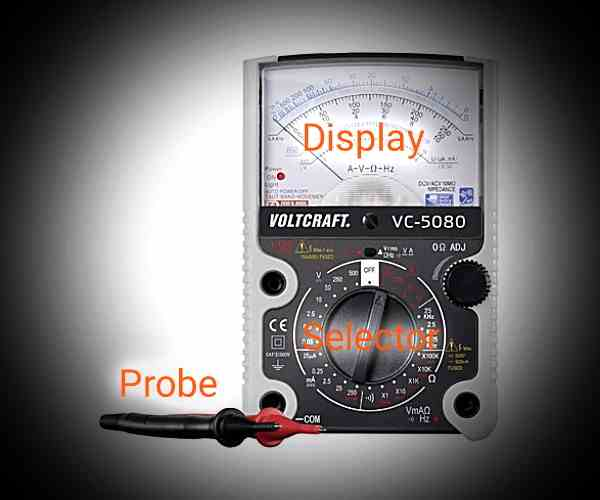# How To Use Analog MultimeterHow To Use Analog Multimeter
How To Use Analog Multitester - Multimeter or which is often referred to as AVO (Ampere Voltase Ohm) meter, is a tool that serves to measure electrical devices about the electric current, voltage and resistance (resistance) in the unit that has been set.

In using multimeter, we must know what units will be measured. Do not get wrong setting that causes damage to multimeter. For example, we will measure the electric current, but we are instead setting it to the barrier panel.

Based on the read view, multimeter is divided into two, namely analog multimeter (needle) and digital multimeter (number). This difference between analog and digital multimeters is seen from the reading screen. Where an analog multimeter uses needles as its measuring instrument, while the digital multimeter is more sophisticated and modern, using a numerical unit.

With the advancement of technology, it turns out that the use of multimeter tool is also progressing its sophistication. In addition to checking the electric current, the voltage and resistance, it is now also able to measure the capacitance, frequency and induction in unit units as well. And here are some of the multimeter functions that are often traded, among others:

• Voltage Measurement Unit (AC and DC Voltage)

• Unit Measurement Ampere (Electric Current)

• OHM Measurement Unit (Resistance)

• Hertz Measurement Unit (Frequency)

• Henry Unit of Measurement (Inductance)

• Diode Measurements

• Transistor Measurement

#### Analog Multimeter Parts And Their Functions

For electricians, the electrical technician must have understood correctly about the parts in the multimeter tool. However, for beginners who are not yet familiar with the multimeter component, little will be explained about the parts of the multimeter that are mandatory to know. And those sections are:

1. Display/Screen

The display or screen is part of the multimeter tool that acts as a measurement result viewer. This display can be either a needle or a number display, depending on the type of multimeter as mentioned above.

2. Selector Switch (Knop Pointer)

The selector switch or pointer knob is the part that serves to direct the unit units to be used for measurement. Suppose we will measure the current voltage of State Electricity Company, then the selector should be directed to the unit of ACV (AC Voltage) units. Do not be wrong in directing, because it will cause damage to the multimeter tool.

3. Probe (Cable Gauge)

A probe or gauge cable is a part used to connect a multimeter with the device to be measured. These cables are generally red and black to make it easy to distinguish which paths between positive (+) and negative (-), especially in the DC measurements of voltage and DC Ampere that require polarity.

### How To Use Analog Multimeter

The following are basic instructions on how to use and read the size of a multimeter for measuring voltage (voltage), electric current (ampere), and the resistance (ohm) in the electric circuit.

#### 1. How To Use Multimeter To Measure AC Voltage (ACV)

• Turn the selector switch in the direction of ACV ~.

• Adjust the selector switch to the largest size.

• Connect the probe to the device to be measured.

• For the AC voltage measurement, the probe has no polarity so it is free to plug (determine) the red (+) and black (-) wires on the device to be measured.

• Look at the size results on the multimeter display. If it turns out that the tension is small, then turn the selector switch to a more keci size in order to get more accurate results. Examples of devices with AC voltage, PLN electricity and Genset.

#### 2. How To Use Multimeter To Measure DC Voltage (DCV)

• Turn the selector switch in the direction of DCV.

• Adjust the selector switch to the largest size.

• Connect the probe to the device to be measured.

• For the DC voltage measurements, set the red cable to the positive (+) terminal and the black cable to the negative terminal (-). Do not turn upside down.

• Look at the size results on the multimeter display. If it turns out that the tension is small, then turn the selector switch to a more keci size in order to get more accurate results.
Examples of devices that have a DC voltage are batteries (ACCU) and power supply DC.

#### 3. How To Use Multimeter To Measure Electric Current (DCA)

• Spin the selector switch towards the DCA.

• Set the largest size of the current range to avoid damage to the multimeter. Because if the measured current exceeds the selected size, a multimeter (fuse) will break.

• Connect the black cord in one section to be measured by the electrical current. And the red cable on one of the other parts. With the record should be created series set between voltage source with multimeter. Because the measured is the electric current. Different from measuring voltages and barriers.

• Then look at the results on the multimeter display.

#### 4. How To Use Multimeter To Measure Barriers (ᘯ)

• Turn the selector switch in the direction of the barrier (ᘯ).

• Adjust the selector switch to the largest size. It usually has an X as the result of its calibration.

• Connect the probe to the component to be measured. For measuring barriers, the probe has no polarity so it is free to plug in (specify) the red (+) and black (-) wires on the device to be measured. The multimeter position and the obstacles to be measured must be parallel.

• Look at the size result on the display. If it turns out the resistance is too small, turn the selector switch to the other X to get a more accurate result.

That's a review of how to use analog multimeter and read how to read the results. Hopefully it can be helpful.